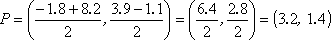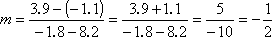Search

The Midpoint Formula (page 2 of 2)

• Is y = 2x  4.9 a bisector of the line segment with endpoints at (1.8, 3.9) and
(8.2, 1.1)?

If I just graph this, it's going to look like the answer is "yes". But I have to remember that a picture can suggest an answer, it can give me an idea of what is going on, but only the algebra can give me an exact answer. So I'll need to find the midpoint, and then see if the midpoint is actually a point on the given line. First, I'll apply the Midpoint Formula:Now I'll check to see if this point is on the line:

y = 2x  4.9
y = 2(3.2)  4.9 = 6.4  4.9 = 1.5

But I needed y to equal 1.4, so this line is close to being a bisector (as a picture would indicate), but it is not exactly a bisector (as the algebra proves). So the answer is "No, this is not a bisector."

• Find the perpendicular bisector of the line segment with endpoints at (1.8, 3.9) and (8.2, 1.1).   Copyright © Elizabeth Stapel 2000-2011 All Rights Reserved

This is a multi-part problem, and is actually typical of problems you will probably encounter at some point when you're learning about straight lines. This is an example of a question where you'll be expected to remember the Midpoint Formula from however long ago you last saw it in class. Don't be surprised if you see this kind of question on a test! Here's how to answer it:

First, I need to find the midpoint, since any bisector, perpendicular or otherwise, must pass through the midpoint. I'll apply the Midpoint Formula:Now I need to find the slope of the line segment. I need this slope in order to find the perpendicular slope. I'll apply the Slope Formula:The perpendicular slope (for my perpendicular bisector) is the negative reciprocal of the slope of the line segment. Remember that "negative reciprocal" means "flip it, and change the sign". Then the slope of the perpendicular bisector will be + 2/1 = 2. With the slope and a point (the midpoint, in this case), I can find the equation of the line:

y  1.4 = 2(x  3.2)
y  1.4 = 2x  6.4
y = 2x  6.4 + 1.4
y = 2x  5

• Find the center of the circle with a diameter having endpoints at (4, 3) and (0, 2).

Since the center is at the midpoint of any diameter, I need to find the midpoint of the two given endpoints:

( [4 + 0]/2 , [3 + 2]/2 ) = ( 4/2 , 5/2 ) = (2, 2.5)

These examples really are fairly typical. You will have some simple "plug-n-chug" problems when the concept is first introduced, and then later, out of the blue, they'll hit you with the concept again, except it will be buried in some other type of problem. I'm telling you this now, so you'll know to remember the Formula for later.

You can use the Mathway widget below to practice finding the midpoint of two points. Try the entered exercise, or enter your own exercise. Then click the "paper-airplane" button to compare your answer to Mathway's.

Please accept "preferences" cookies in order to enable this widget.

(Clicking on "Tap to view steps" on the widget's answer screen will take you to the Mathway site for a paid upgrade.)

<< Previous  Top  |  1 | 2  |  Return to Index

 Cite this article as: Stapel, Elizabeth. "The Midpoint Formula: worked Examples." Purplemath. Available from     https://www.purplemath.com/modules/midpoint2.htm. Accessed [Date] [Month] 2016

Study Skills Survey

Tutoring from Purplemath
Find a local math tutor

 Copyright © 2021  Elizabeth Stapel   |   About   |   Terms of Use   |   Linking   |   Site Licensing Contact Us